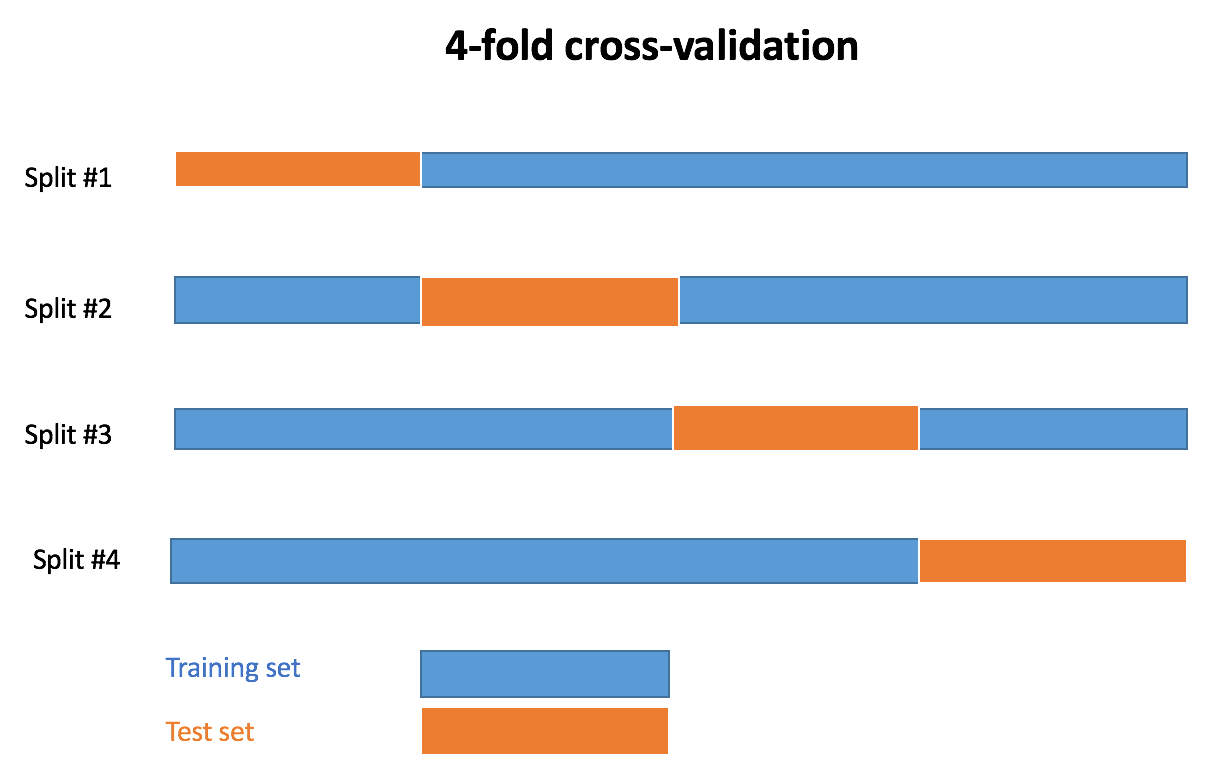Want to share your content on R-bloggers? click here if you have a blog, or here if you don't.

As seen last week in a post on grid search cross-validation, `crossval` contains generic functions for statistical/machine learning cross-validation in R. A 4-fold cross-validation procedure is presented below:In this post, I present some examples of use of `crossval` on a linear model, and on the popular `xgboost` and `randomForest` models. The error measure used is Root Mean Squared Error (RMSE), and is currently the only choice implemented.

## Installation

From Github, in R console:

``````devtools::install_github("thierrymoudiki/crossval")
``````

## Demo

We use a simulated dataset for this demo, containing 100 examples, and 5 explanatory variables:

``````# dataset creation
set.seed(123)
n <- 100 ; p <- 5
X <- matrix(rnorm(n * p), n, p)
y <- rnorm(n)
``````

### Linear model

• `X` contains the explanatory variables
• `y` is the response
• `k` is the number of folds in k-fold cross-validation
• `repeats` is the number of repeats of the k-fold cross-validation procedure

Linear model example:

``````crossval::crossval_ml(x = X, y = y, k = 5, repeats = 3)
``````
``````## \$folds
##         repeat_1  repeat_2  repeat_3
## fold_1 0.8987732 0.9270326 0.7903096
## fold_2 0.8787553 0.8704522 1.2394063
## fold_3 1.0810407 0.7907543 1.3381991
## fold_4 1.0594537 1.1981031 0.7368007
## fold_5 0.7593157 0.8913229 0.7734180
##
## \$mean
##  0.9488758
##
## \$sd
##  0.1902999
##
## \$median
##  0.8913229
``````

Linear model example, with validation set:

``````crossval::crossval_ml(x = X, y = y, k = 5, repeats = 3, p = 0.8)
``````
``````## \$folds
##                    repeat_1  repeat_2  repeat_3
## fold_training_1   1.1256933 0.9144503 0.9746044
## fold_validation_1 0.9734644 0.9805410 0.9761265
## fold_training_2   1.0124938 0.9652489 0.7257494
## fold_validation_2 0.9800293 0.9577811 0.9631389
## fold_training_3   0.7695705 1.0091999 0.9740067
## fold_validation_3 0.9753250 1.0373943 0.9863062
## fold_training_4   1.0482233 0.9194648 0.9680724
## fold_validation_4 0.9984861 0.9596531 0.9742874
## fold_training_5   0.9210179 1.0455006 0.9886350
## fold_validation_5 1.0126038 0.9658146 0.9658412
##
## \$mean_training
##  0.9574621
##
## \$mean_validation
##  0.9804529
##
## \$sd_training
##  0.1018837
##
## \$sd_validation
##  0.02145046
##
## \$median_training
##  0.9740067
##
## \$median_validation
##  0.975325
``````

### Random Forest

randomForest example:

``````
require(randomForest)

# fit randomForest with mtry = 4

crossval::crossval_ml(x = X, y = y, k = 5, repeats = 3,
fit_func = randomForest::randomForest, predict_func = predict,
packages = "randomForest", fit_params = list(mtry = 4))
``````
``````## \$folds
##         repeat_1  repeat_2  repeat_3
## fold_1 0.9820183 0.9895682 0.8752296
## fold_2 0.8701763 0.8771651 1.2719188
## fold_3 1.1869986 0.7736392 1.3521407
## fold_4 1.0946892 1.1204090 0.7100938
## fold_5 0.9847612 1.0565001 0.9194678
##
## \$mean
##  1.004318
##
## \$sd
##  0.1791315
##
## \$median
##  0.9847612
``````

`randomForest` with parameter `mtry` = 4, and a validation set:

``````
crossval::crossval_ml(x = X, y = y, k = 5, repeats = 2, p = 0.8,
fit_func = randomForest::randomForest, predict_func = predict,
packages = "randomForest", fit_params = list(mtry = 4))
``````
``````## \$folds
##                    repeat_1  repeat_2
## fold_training_1   1.0819863 0.9096807
## fold_validation_1 0.8413615 0.8415839
## fold_training_2   0.9507086 1.0014771
## fold_validation_2 0.5631285 0.6545253
## fold_training_3   0.7020669 0.9632402
## fold_validation_3 0.5090071 0.9129895
## fold_training_4   0.8932151 1.0315366
## fold_validation_4 0.8299454 0.7147867
## fold_training_5   0.9158418 1.1093461
## fold_validation_5 0.6438410 0.7644071
##
## \$mean_training
##  0.9559099
##
## \$mean_validation
##  0.7275576
##
## \$sd_training
##  0.1151926
##
## \$sd_validation
##  0.133119
##
## \$median_training
##  0.9569744
##
## \$median_validation
##  0.7395969
``````

### xgboost

In this case, the response and covariates are named ‘label’ and ‘data’. So (for now), we do this:

``````# xgboost example -----

require(xgboost)

f_xgboost <- function(x, y, ...) xgboost::xgboost(data = x, label = y, ...)
``````

Fit `xgboost` with `nrounds` = 10:

``````

crossval::crossval_ml(x = X, y = y, k = 5, repeats = 3,
fit_func = f_xgboost, predict_func = predict,
packages = "xgboost", fit_params = list(nrounds = 10,
verbose = FALSE))
``````
``````## \$folds
##         repeat_1  repeat_2  repeat_3
## fold_1 0.9487191 1.2019850 0.9160024
## fold_2 0.9194731 0.8990731 1.2619773
## fold_3 1.2775092 0.7691470 1.3942022
## fold_4 1.1893053 1.1250443 0.7173760
## fold_5 1.1200368 1.1686622 0.9986680
##
## \$mean
##  1.060479
##
## \$sd
##  0.1965465
##
## \$median
##  1.120037
``````

Fit `xgboost` with `nrounds = 10, and validation set:

``````crossval::crossval_ml(x = X, y = y, k = 5, repeats = 2, p = 0.8,
fit_func = f_xgboost, predict_func = predict,
packages = "xgboost", fit_params = list(nrounds = 10,
verbose = FALSE))
``````
``````## \$folds
##                    repeat_1  repeat_2
## fold_training_1   1.1063607 1.0350719
## fold_validation_1 0.7891655 1.0025217
## fold_training_2   1.0117042 1.1723135
## fold_validation_2 0.4325200 0.5050369
## fold_training_3   0.7074600 1.0101371
## fold_validation_3 0.1916094 0.9800865
## fold_training_4   0.9131272 1.2411424
## fold_validation_4 0.8998582 0.7521359
## fold_training_5   0.9462418 1.0543695
## fold_validation_5 0.5432650 0.6850912
##
## \$mean_training
##  1.019793
##
## \$mean_validation
##  0.678129
##
## \$sd_training
##  0.147452
##
## \$sd_validation
##  0.2600431
##
## \$median_training
##  1.023388
##
## \$median_validation
##  0.7186136
``````

Note: I am currently looking for a gig. You can hire me on Malt or send me an email: thierry dot moudiki at pm dot me. I can do descriptive statistics, data preparation, feature engineering, model calibration, training and validation, and model outputs’ interpretation. I am fluent in Python, R, SQL, Microsoft Excel, Visual Basic (among others) and French. My résumé? Here!